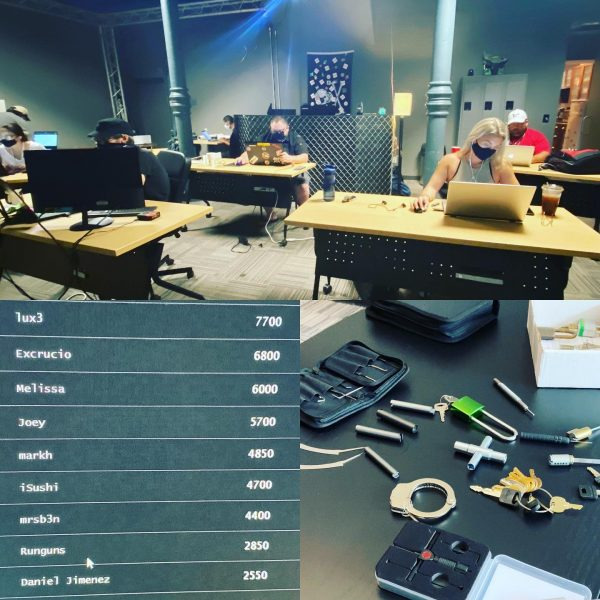Categories

# A more Pythonic approach to one of my “Capture the Flag” solutionsIn my article about the Capture the Flag at The Undercroft in which I recently participated, I wrote about my solution to this particular challenge:

`0010111 00001111 00010111, 00011001 00001111 10101 00000001 00010010 00000101 00010010 00001001 00000111 00001000 00010100`

(Make sure to use the comma, and spaces correctly)

The first part of my solution was turning those numbers into a list. Copy the numbers into a text editor, stick `0b` in front of each one, and then turn the sequence into a Python list:

`numbers = [0b0010111, 0b00001111, 0b00010111, 0b00011001, 0b00001111, 0b10101, 0b00000001, 0b00010010, 0b00000101, 0b00010010, 0b00001001, 0b00000111, 0b00001000, 0b00010100]`

Paste the list into a Python REPL and then display its contents to see the numbers in decimal:

```>>> numbers
[23, 15, 23, 25, 15, 21, 1, 18, 5, 18, 9, 7, 8, 20]```

The next step is to convert those numbers into letters. Once again, the Unicode/ASCII value for “A” is 65, so the trick is to add 64 to each number and convert the resulting number into a character.

Here’s how I did that:

```>>> characters = map(lambda number: chr(number + 64), numbers)
>>> list(characters)
['W', 'O', 'W', 'Y', 'O', 'U', 'A', 'R', 'E', 'R', 'I', 'G', 'H', 'T']```

I could’ve gone super-functional and done it in one line:

```>>> list(map(lambda number: chr(number + 64), numbers))
['W', 'O', 'W', 'Y', 'O', 'U', 'A', 'R', 'E', 'R', 'I', 'G', 'H', 'T']```

Between `lambda` and `map()`, there’s a whole lot of functional programming concepts to solve a relatively simple problem.

I could write a whole article — and I probably should — based on just that single line of code, but in the meantime, I thought I’d post an easier, more Pythonic solution.

This simpler solution uses good ol’ list comprehensions:

```>>> characters = [chr(number + 64) for number in numbers]
>>> characters
['W', 'O', 'W', 'Y', 'O', 'U', 'A', 'R', 'E', 'R', 'I', 'G', 'H', 'T']```

Most programming languages don’t have list comprehensions. In those languages, if you want to perform some operation on every item in an array, you use a mapping function, typically named `map()`, but sometimes `collect()` or `select()`.

Hence my original solution with `lambda` and `map()` — it’s force of habit from working in JavaScript, Kotlin, Ruby, and Swift, which don’t have Python’s nifty list comprehensions.